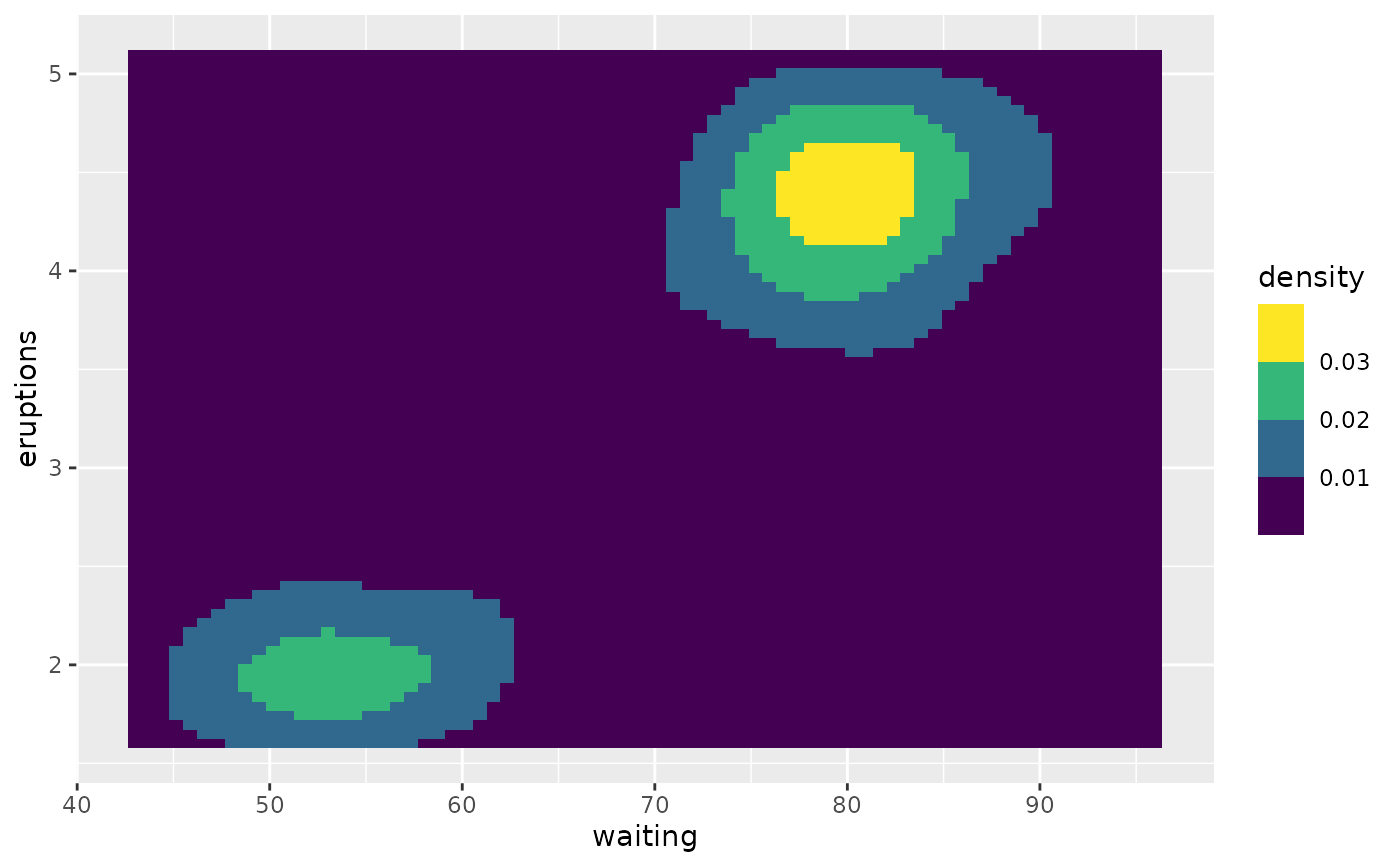The viridis scales provide colour maps that are perceptually uniform in both colour and black-and-white. They are also designed to be perceived by viewers with common forms of colour blindness. See also https://bids.github.io/colormap/.

## Usage

scale_colour_viridis_d(
...,
alpha = 1,
begin = 0,
end = 1,
direction = 1,
option = "D",
aesthetics = "colour"
)

scale_fill_viridis_d(
...,
alpha = 1,
begin = 0,
end = 1,
direction = 1,
option = "D",
aesthetics = "fill"
)

scale_colour_viridis_c(
...,
alpha = 1,
begin = 0,
end = 1,
direction = 1,
option = "D",
values = NULL,
space = "Lab",
na.value = "grey50",
guide = "colourbar",
aesthetics = "colour"
)

scale_fill_viridis_c(
...,
alpha = 1,
begin = 0,
end = 1,
direction = 1,
option = "D",
values = NULL,
space = "Lab",
na.value = "grey50",
guide = "colourbar",
aesthetics = "fill"
)

scale_colour_viridis_b(
...,
alpha = 1,
begin = 0,
end = 1,
direction = 1,
option = "D",
values = NULL,
space = "Lab",
na.value = "grey50",
guide = "coloursteps",
aesthetics = "colour"
)

scale_fill_viridis_b(
...,
alpha = 1,
begin = 0,
end = 1,
direction = 1,
option = "D",
values = NULL,
space = "Lab",
na.value = "grey50",
guide = "coloursteps",
aesthetics = "fill"
)

## Arguments

...

Other arguments passed on to discrete_scale(), continuous_scale(), or binned_scale() to control name, limits, breaks, labels and so forth.

alpha

The alpha transparency, a number in [0,1], see argument alpha in hsv.

begin, end

The (corrected) hue in [0,1] at which the color map begins and ends.

direction

Sets the order of colors in the scale. If 1, the default, colors are ordered from darkest to lightest. If -1, the order of colors is reversed.

option

A character string indicating the color map option to use. Eight options are available:

• "magma" (or "A")

• "inferno" (or "B")

• "plasma" (or "C")

• "viridis" (or "D")

• "cividis" (or "E")

• "rocket" (or "F")

• "mako" (or "G")

• "turbo" (or "H")

aesthetics

Character string or vector of character strings listing the name(s) of the aesthetic(s) that this scale works with. This can be useful, for example, to apply colour settings to the colour and fill aesthetics at the same time, via aesthetics = c("colour", "fill").

values

if colours should not be evenly positioned along the gradient this vector gives the position (between 0 and 1) for each colour in the colours vector. See rescale() for a convenience function to map an arbitrary range to between 0 and 1.

space

colour space in which to calculate gradient. Must be "Lab" - other values are deprecated.

na.value

Missing values will be replaced with this value.

guide

A function used to create a guide or its name. See guides() for more information.

Other colour scales: scale_alpha(), scale_colour_brewer(), scale_colour_continuous(), scale_colour_gradient(), scale_colour_grey(), scale_colour_hue(), scale_colour_steps()

## Examples

# viridis is the default colour/fill scale for ordered factors
set.seed(596)
dsamp <- diamonds[sample(nrow(diamonds), 1000), ]
ggplot(dsamp, aes(carat, price)) +
geom_point(aes(colour = clarity))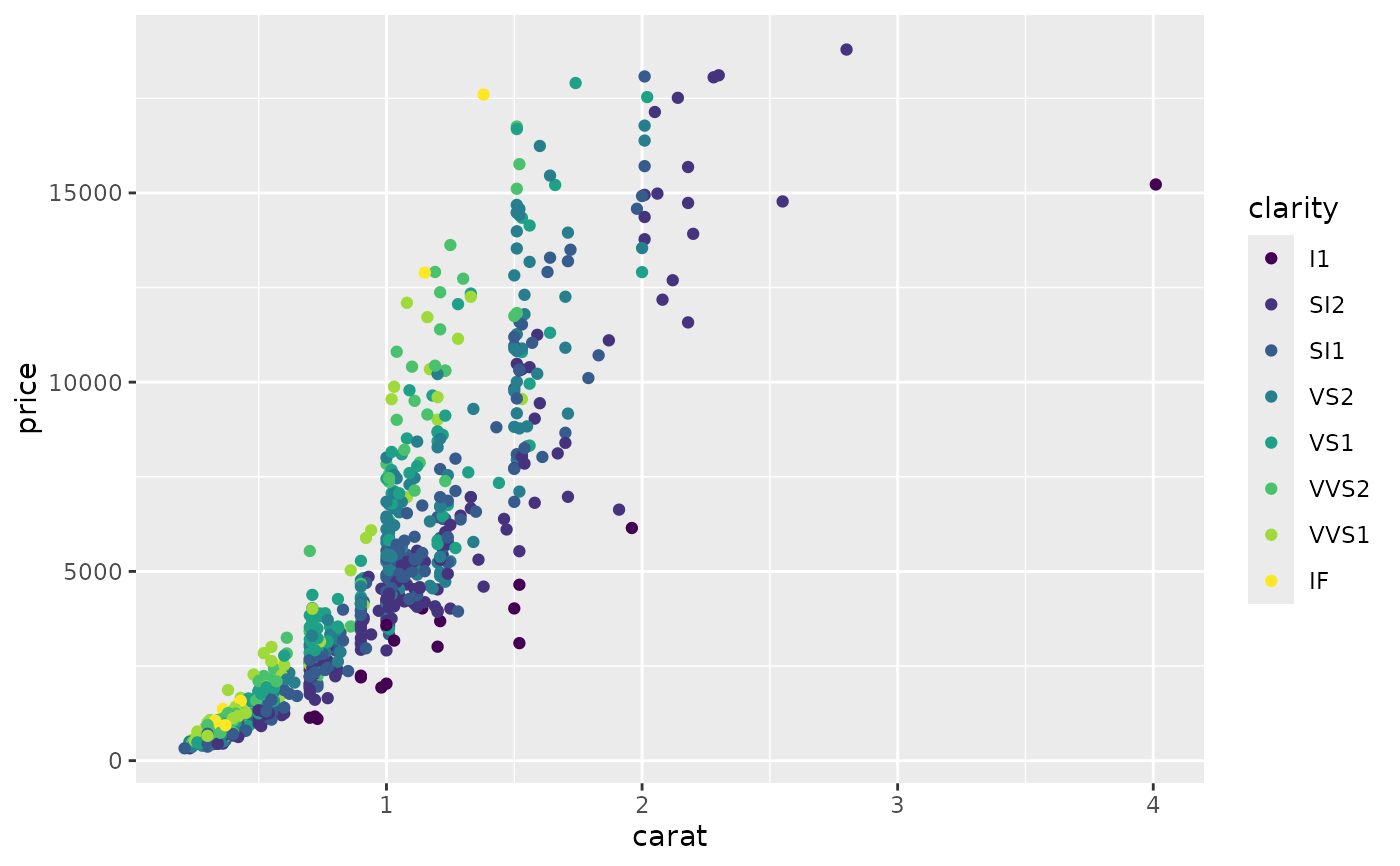# Use viridis_d with discrete data
txsamp <- subset(txhousing, city %in%
c("Houston", "Fort Worth", "San Antonio", "Dallas", "Austin"))
(d <- ggplot(data = txsamp, aes(x = sales, y = median)) +
geom_point(aes(colour = city)))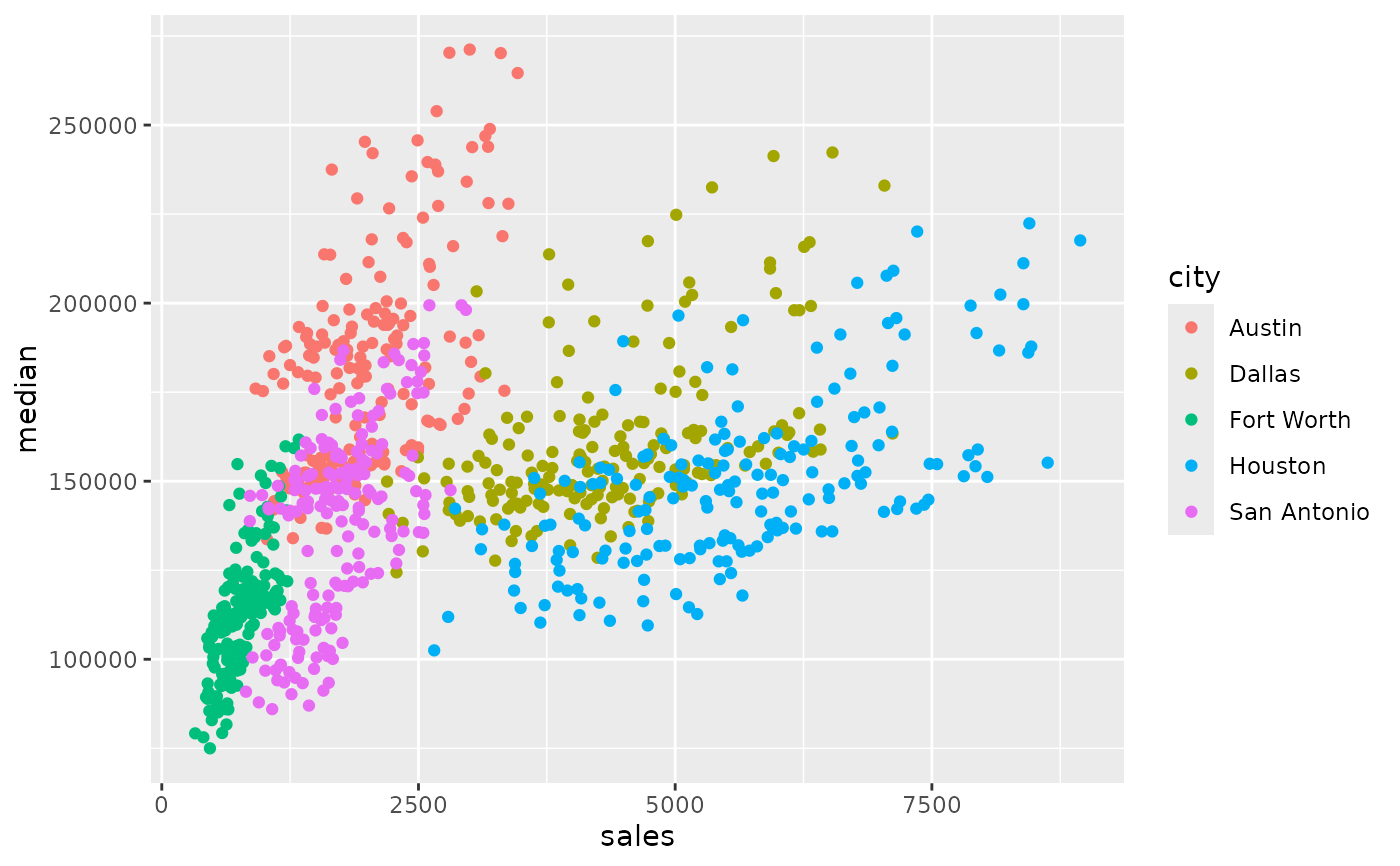d + scale_colour_viridis_d()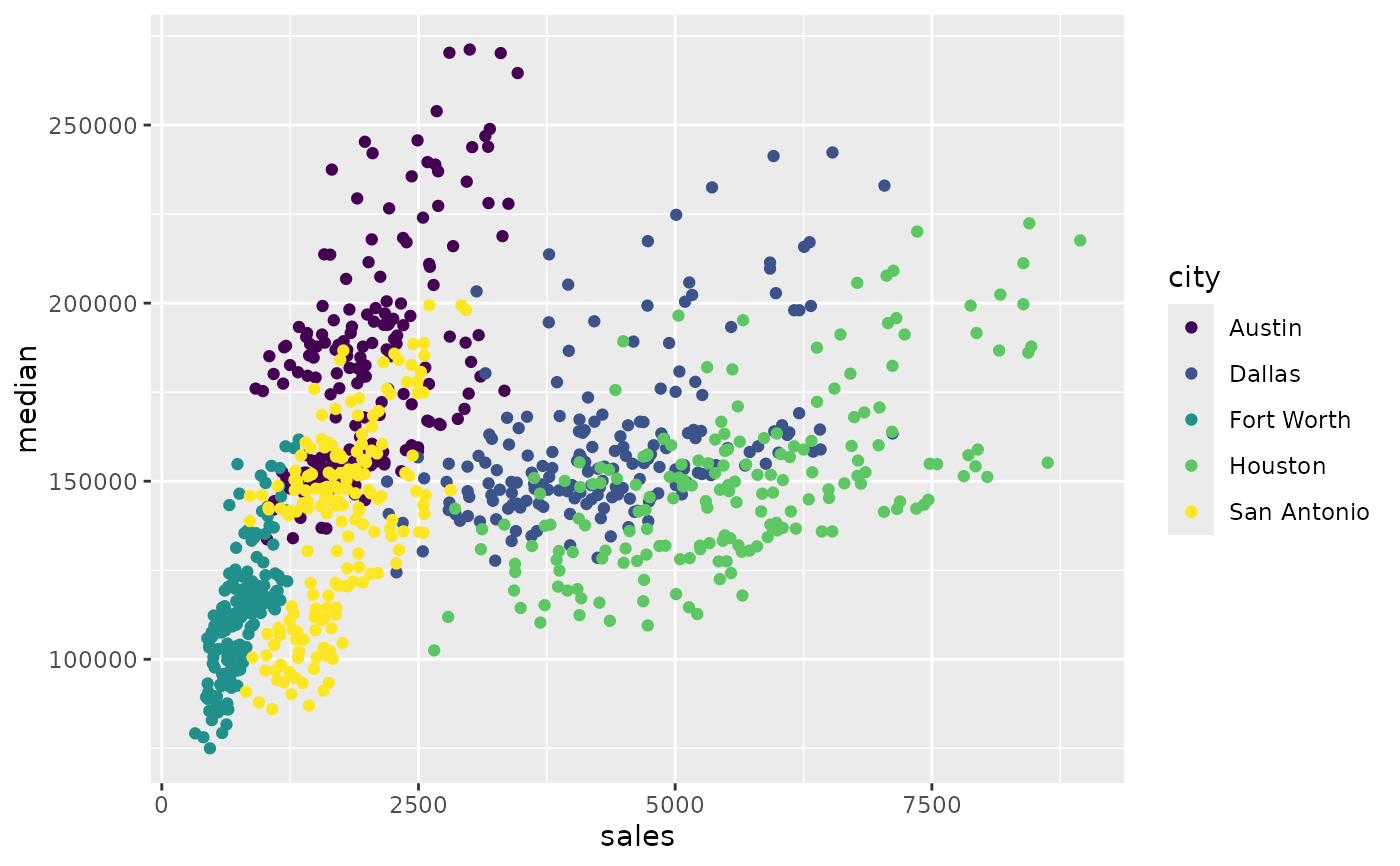# Change scale label
d + scale_colour_viridis_d("City\nCenter")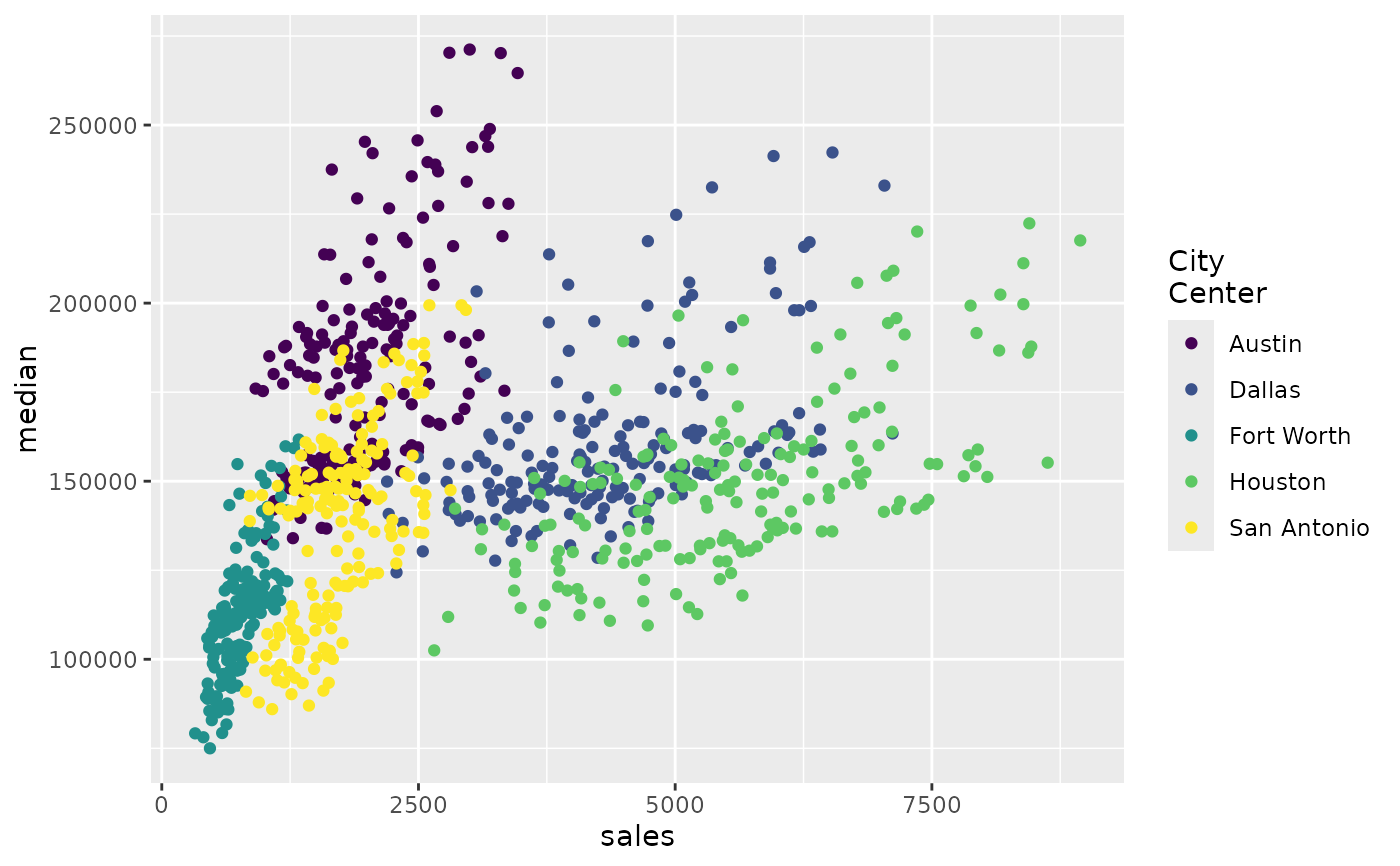# Select palette to use, see ?scales::viridis_pal for more details
d + scale_colour_viridis_d(option = "plasma")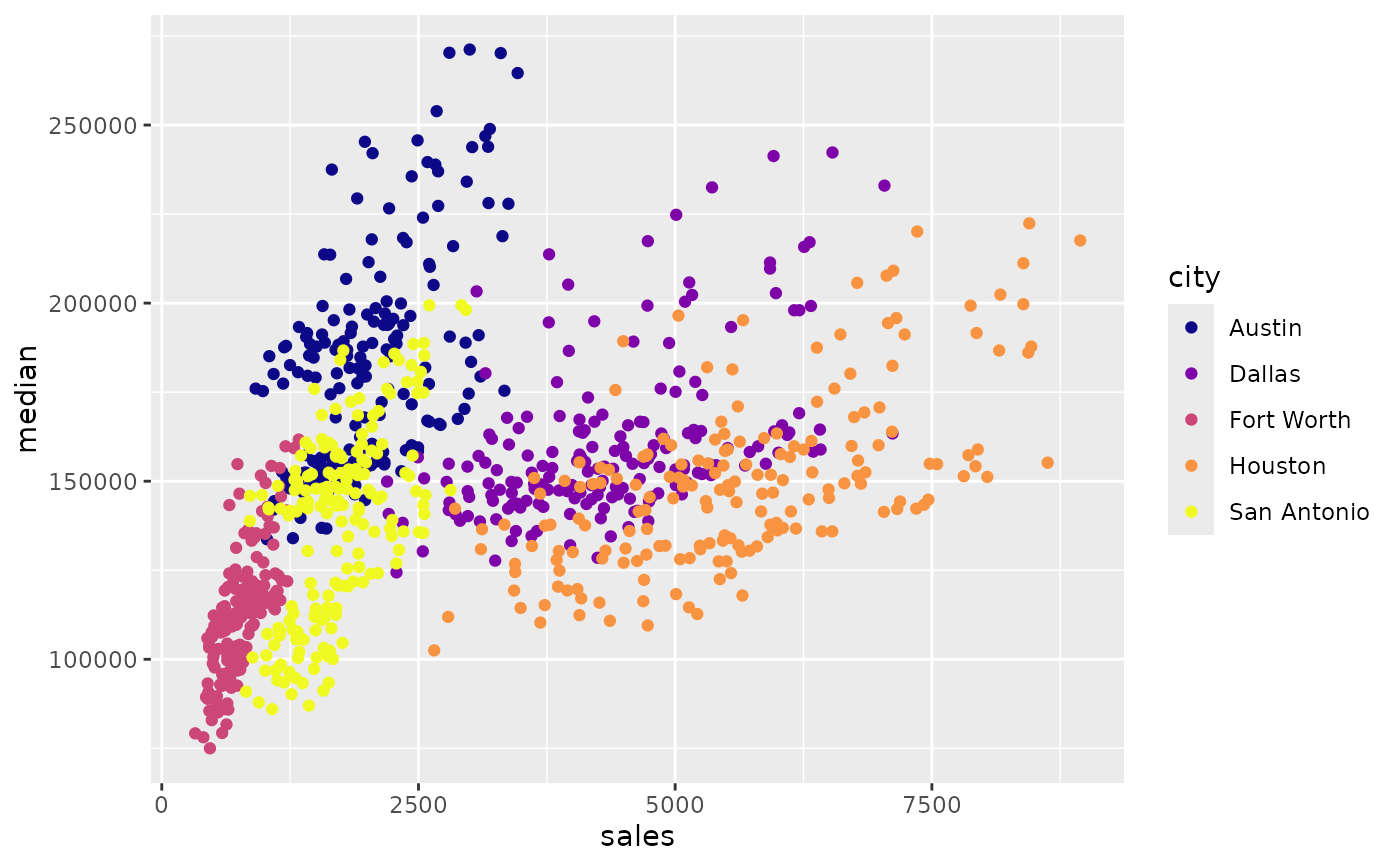d + scale_colour_viridis_d(option = "inferno")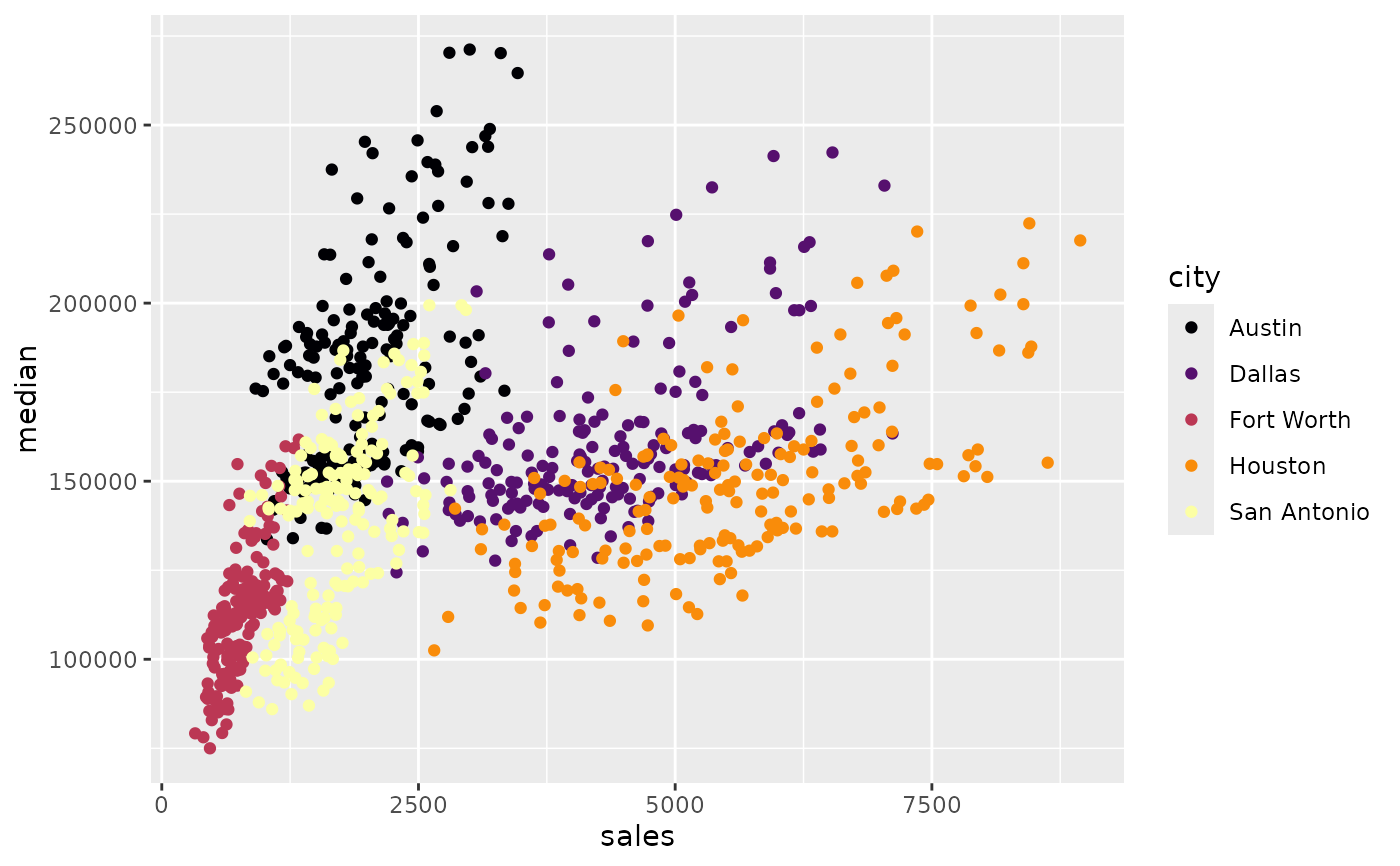# scale_fill_viridis_d works just the same as
# scale_colour_viridis_d but for fill colours
p <- ggplot(txsamp, aes(x = median, fill = city)) +
geom_histogram(position = "dodge", binwidth = 15000)
p + scale_fill_viridis_d()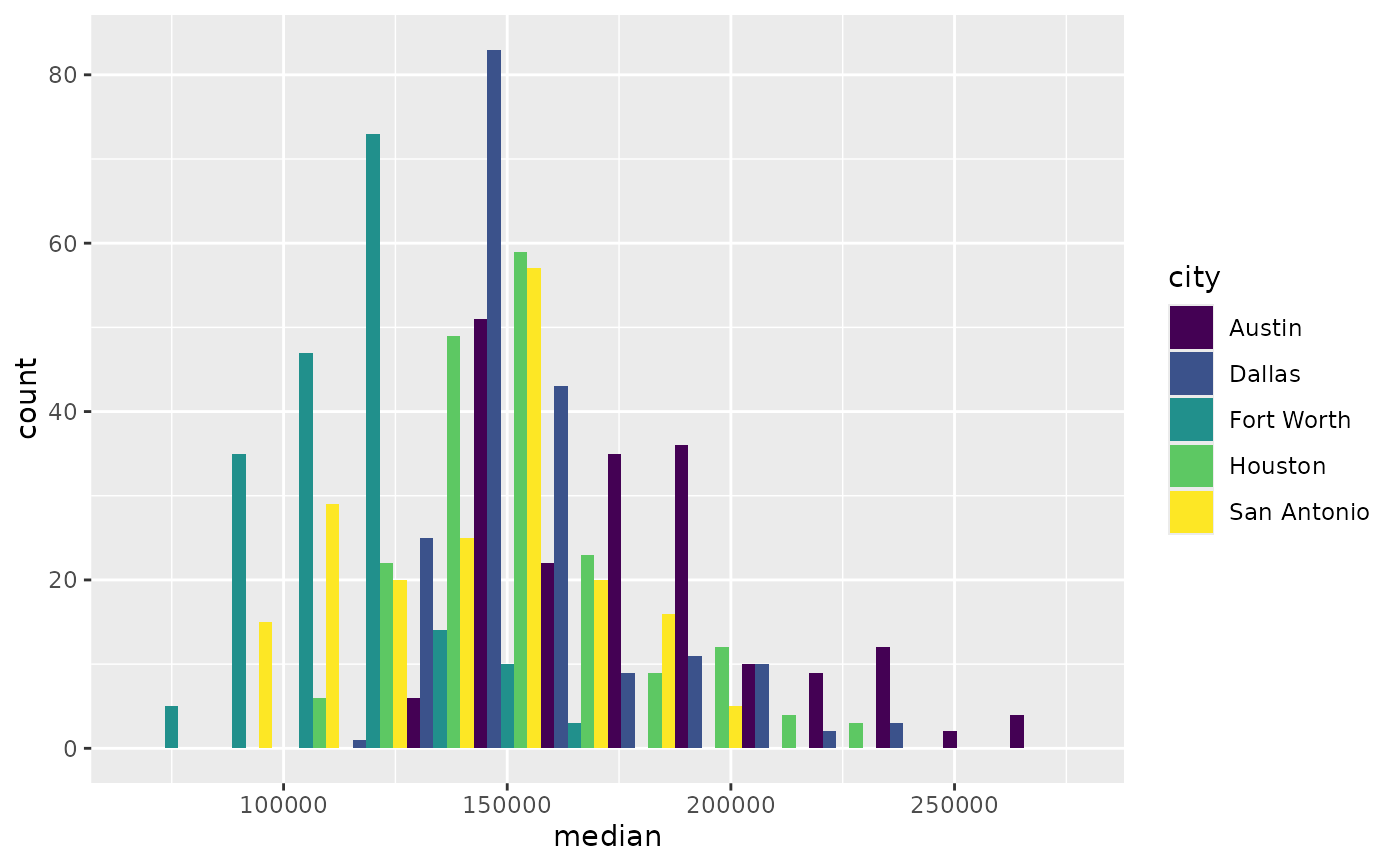# the order of colour can be reversed
p + scale_fill_viridis_d(direction = -1)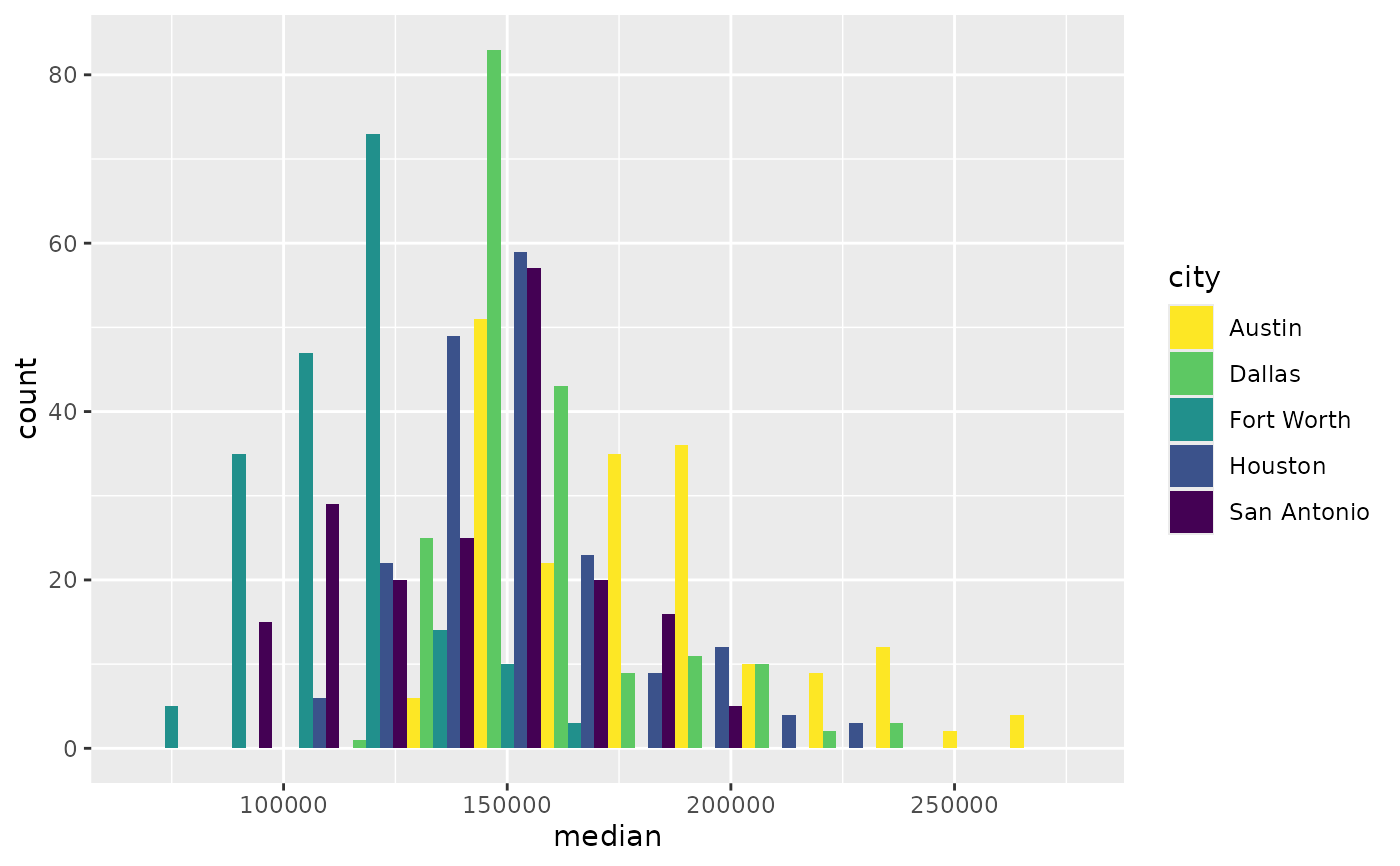# Use viridis_c with continous data
(v <- ggplot(faithfuld) +
geom_tile(aes(waiting, eruptions, fill = density)))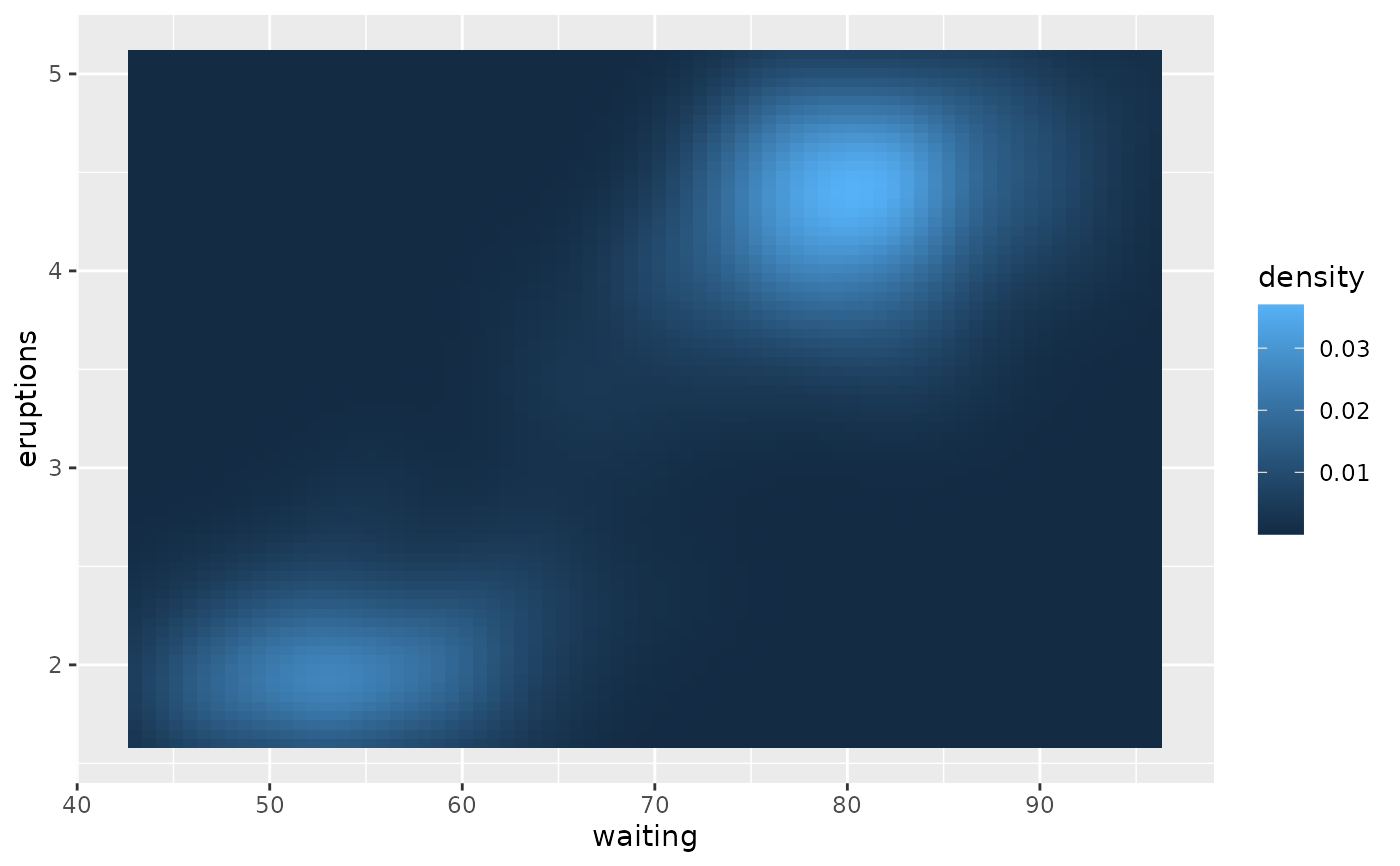v + scale_fill_viridis_c()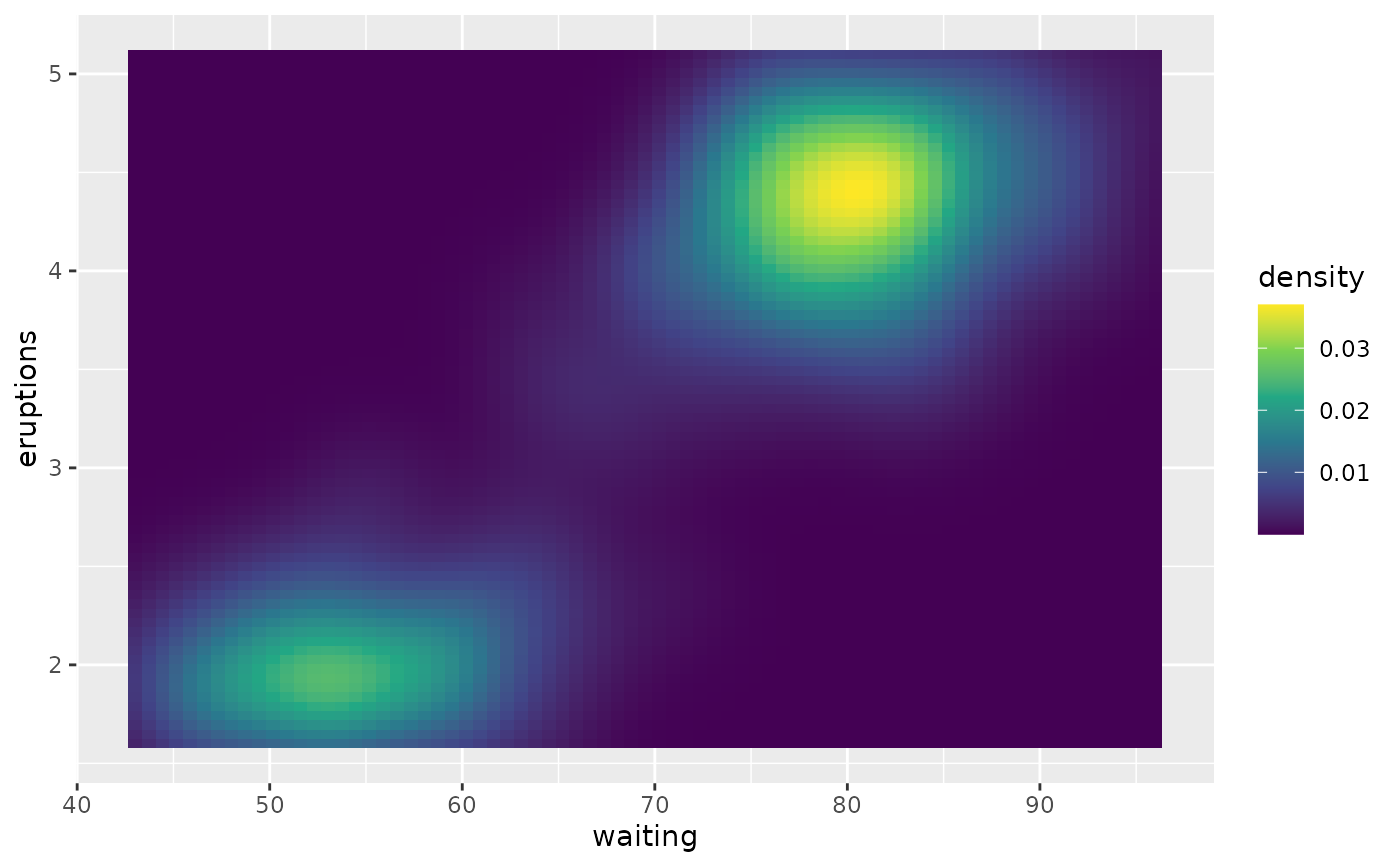v + scale_fill_viridis_c(option = "plasma")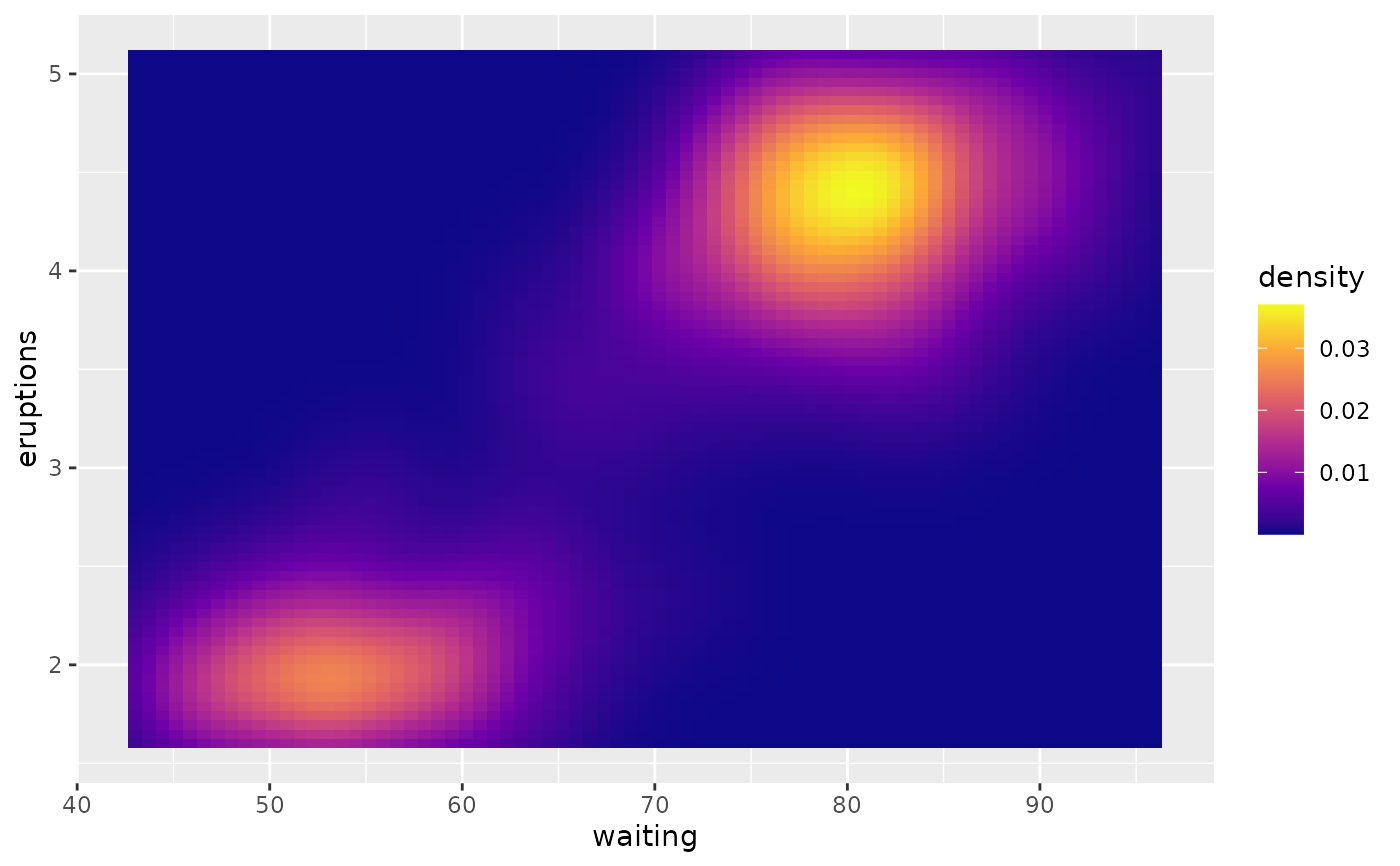# Use viridis_b to bin continuous data before mapping
v + scale_fill_viridis_b()